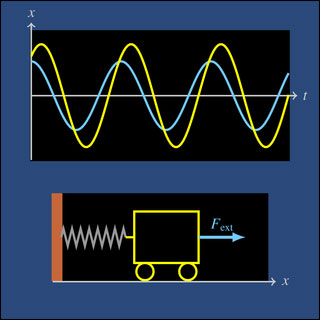18.03SC | Fall 2011 | Undergraduate

# Differential Equations

## Unit II: Second Order Constant Coefficient Linear Equations

« Previous | Next »Periodic response of a second order system. Modeled on the MIT mathlet Amplitude and Phase: Second Order I.

In this unit we learn how to solve constant coefficient second order linear differential equations, and also how to interpret these solutions when the DE is modeling a physical system. The language and ideas we introduced for first order linear constant coefficient DE’s carry forward to the second order case—in particular, the breakdown into the homogeneous and inhomogeneous cases; the input-response language for the inhomogeneous case; and the general form of solutions as

x = xh + xp'

where xh is the general solution to the homogeneous equation, and xp is any particular solution to the inhomogeneous equation.

We first learn how to solve the homogeneous equation

ax" + bx’ + cx = 0

As in the first order case, the solutions will be exponential functions. In the second order case, however, the exponential functions can be either real or complex, so that we need to use the complex arithmetic and complex exponentials we developed in the last unit.

For the second order inhomogeneous DE

ax" + bx’ + cx = ƒ(t)

we will concentrate much of our attention on the important case of a sinusoidal signal ƒ(t) = B cos(ωt), and again see how we can simplify the calculations and achieve a better understanding of the solution methods and the solutions themselves by using complex exponentials and arithmetic.

We will run an interpretation of the results as the behavior of a physical system in parallel with the mathematical methods and formulas, in order to have a concrete way to understand and visualize what these results mean. We will use a spring-mass mechanical system for the most part, since it is easy to picture. At the end of the unit we will also explore the application of this theory to electrical circuits, which is another very important use of these mathematical methods.

« Previous | Next »

## Course Info

Fall 2011
##### Learning Resource Types
Lecture Videos
Recitation Videos
Exams with Solutions
Simulations
Lecture Notes
Problem Sets with Solutions